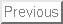Next: Introduction

Probabilistic ancestral sequences and Multiple alignments

Gaston H. Gonnet and Steven A. Benner
Informatik - Organic Chemistry
E.T.H.
Zurich, Switzerland

Abstract:

An evolutionary configuration (EC) is a set of aligned sequences of characters (possibly representing amino acids, DNA, RNA or natural language). We define the probability of an EC, based on a given phylogenetic tree and give an algorithm to compute this probability efficiently. From these probabilities, we can compute the most likely sequence at any place in the phylogenetic tree, or its probability profile. The probability profile at the root of the tree is called the probabilistic ancestral sequence. By computing the probability of an EC, we can find by dynamic programming alignments over two subtrees. This gives an algorithm for computing multiple alignments. These multiple alignments are maximum likelihood, and are a compatible generalization of two sequence alignments.

Gaston Gonnet
1998-07-14